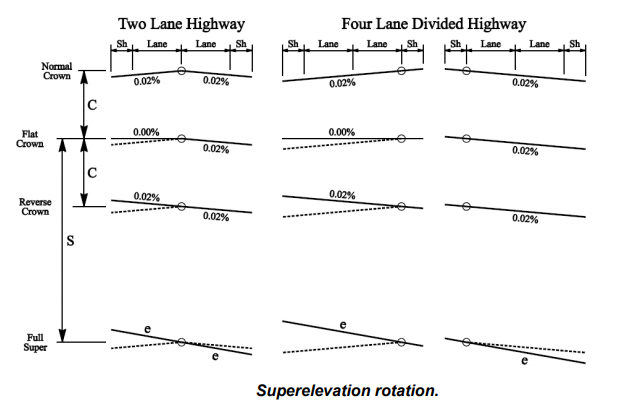### Super-elevation

If you ever drive on a curved road, you would notice that the cross-slope of the road is larger than other portions of the road, as shown in figure no:1. The cross-slope for curves commonly known as superelevation and it is used to counteract the effect of centrifugal force and stabilize the vehicles moving above the curved road. Driving the vehicles above a curve with a small radius will cause a large centrifugal force, which may cause a considerable lateral force on a vehicle, and it can result in losing the control of the vehicle.

Figure 1

Superelevation is the change in road cross-slope. It can be done by increasing the level of the outer road edge, as shown in figure 1. superelevation and transverse friction will act together to stabilize the vehicle traveling on a curved road. Superelevation will allow a safer movement of vehicles at a higher speed compared to a curved road with no superelevation. Superelevation can be calculated using the following equation.

Superelevation=e+f=V^2/127R

Where

e is the rate of superelevation

f is the lateral friction factor

V is the velocity of the vehicle m/s

R is the radius of the curve in m.

We can notice from the above equation that the superelevation disproportional to curve radius. If the road curves increase, the superelevation will decrease. Increasing the radius will reduce the centrifugal forces. On the other, superelevation proportional to design speed. The increase of design speed will require a higher superelevation to counteract the centrifugal force and stabilize the moving vehicles.

Figure no:2 shows the change in the gradual rotation of pavement cross-section. The transition will occur when the road alignment change from straight to curved. The transition of pavement cross-section will be gradual to assure a smooth-riding of road users. We can see in the figure below two types of roads. Undivided and divided highways. In an undivided highway, the rotation occurs at the centerline of the highway while on the divided highway, the rotation occurs at the inner edge of the road. Initially, the pavement cross-section transferred from a normal crown to a flat crown. The next stage will be a reverse crown, where the existing slope of half portion of the highway reversed, as shown in figure no:2. Finally, the pavement rotated gradually to the required superelevation. The transition of road cross-slope will occur at both ends of a circular curve.Figure 2